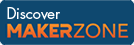Cody

# Problem 2024. Triangle sequence

Created by Tanya Morton in Community

A sequence of triangles is constructed in the following way:

1) the first triangle is Pythagoras' 3-4-5 triangle

2) the second triangle is a right-angle triangle whose second longest side is the hypotenuse of the first triangle, and whose shortest side is the same length as the second longest side of the first triangle

3) the third triangle is a right-angle triangle whose second longest side is the hypotenuse of the second triangle, and whose shortest side is the same length as the second longest side of the second triangle etc.

Each triangle in the sequence is constructed so that its second longest side is the hypotenuse of the previous triangle and its shortest side is the same length as the second longest side of the previous triangle.

What is the area of a square whose side is the hypotenuse of the nth triangle in the sequence?

### Solution Stats

41.02% Correct | 58.98% Incorrect
Last solution submitted on Mar 25, 2019

#### TagsMATLAB and Simulink resources for Arduino, LEGO, and Raspberry Pi